## Biharmonic Equation

The differential equation obtained by applying the Biharmonic Operator and setting to zero.(1)

In Cartesian Coordinates, the biharmonic equation is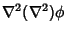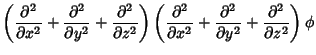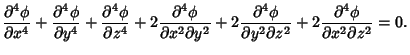(2)

In Polar Coordinates (Kaplan 1984, p. 148)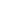(3)

For a radial function, the biharmonic equation becomes(4)

Writing the inhomogeneous equation as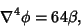(5)

we have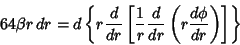(6)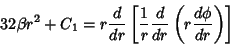(7)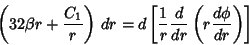(8)(9)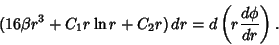(10)

Now use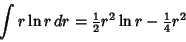(11)

to obtain(12)(13)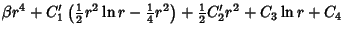(14)

The homogeneous biharmonic equation can be separated and solved in 2-D Bipolar Coordinates.

References